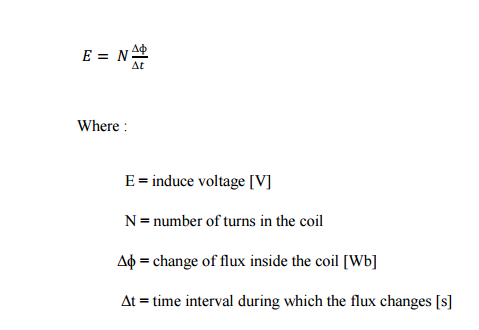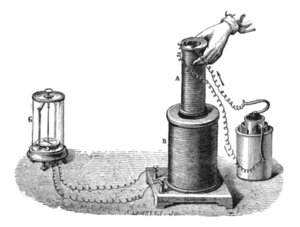# Faraday’s Law of Electromagnetic Induction

According to the Faraday’s law of electromagnetic induction, emf gets induced in the rotor. As the rotor circuit is closed one so, the current starts flowing in the rotor. This currents called the rotor current. This rotor current produces its own flux called rotor flux. Since this flux is produced due to induction principle so, the motor working on this principle got its name as induction motor. Now there are two fluxes one is main flux and another is called rotor flux. These two fluxes produce the desired torque which is required by the motor to rotate.

In 1831, while pursuing his experiment, Michael Faraday made one of most important discoveries in electromagnetism. Now known as Faraday’s Law of electromagnetic induction, it reveals a fundamental relationship between the voltage and flux in a circuit.

a) If the flux linking a loop (or turn) varies as a function of time, a voltage is induce between its terminals.

b) The value of the induce voltage is proportional to the rate of change of flux.

By definition and according to the SI system of units, when the flux inside a loop varies at the rate of 1 Weber per-second, a voltage of 1V is induce between its terminals. Consequently, if the flux varies inside a coil of N turns, the voltage induced is given byFaraday’s law of electromagnetic induction opened the door to a host of practical applications and established the basis of operation of transformers, generator and alternating current motor.Faraday’s experiment showing induction between coils of wire: The liquid battery (right) provides a current which flows through the small coil (A), creating a magnetic field. When the coils are stationary, no current is induced. But when the small coil is moved in or out of the large coil (B), the magnetic flux through the large coil changes, inducing a current which is detected by the galvanometer (G).

error: Content is protected !!